## 19.5. Contouring algorithm

19.5.1. Introduction
19.5.2. CONREC
19.5.3. Algorithm
19.5.4. Example
19.5.5. Subroutine
19.5.6. Example
19.5.7. Note

The contouring algorithm employed within Debrief was originally produced by Paul Bourke back in 1987, for submission to the Byte magazine. Here's the article pretty much verbatim:

### 19.5.1. Introduction

This article introduces a straightforward method of contouring some surface represented as a regular triangular mesh.

Contouring aids in visualizing three dimensional surfaces on a two dimensional medium (on paper or in this case a computer graphics screen).

Two most common applications are displaying topological features of an area on a map or the air pressure on a weather map. In all cases some parameter is plotted as a function of two variables, the longitude and latitude or x and y axis. One problem with computer contouring is the process is usually CPU intensive and the algorithms often use advanced mathematical techniques making them susceptible to error.

### 19.5.2. CONREC

To do contouring in software you need to describe the data surface and the contour levels you want to have drawn. The software given this information must call the algorithm that calculates the line segments that make up a contour curve and then plot these line segments on whatever graphics device is available.

CONREC satisfies the above description, it is relatively simple to implement, very reliable, and does not require sophisticated programming techniques or a high level of mathematics to understand how it works.

The input parameters to the CONREC subroutine are as follows :

• The number of horizontal and vertical data points designated iub and jub.

• The number of contouring levels, nc.

• A one dimensional array z(0:nc-1) that saves as a list of the contour levels in increasing order. (The order of course can be relaxed if the program will sort the levels)

• A two dimensional array d(0:iub,0:jub) that contains the description of the data array to be contoured. Each element of the array is a sample of the surface being studied at a point (x,y)

• Two, one dimensional arrays x(0:iub) and y(0:jub) which contain the horizontal and vertical coordinates of each sample point. This allows for a rectangular grid of samples.

Figure 19.2. Some of the CONREC input parameters.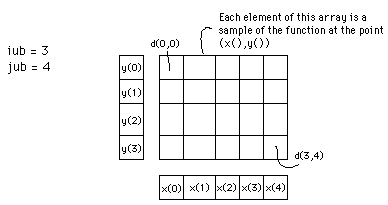The contouring subroutine CONREC does not assume anything about the device that will be used to plot the contours. It instead expects a user written subroutine called VECOUT. CONREC calls VECOUT with the horizontal and vertical coordinates of the start and end coordinates of a line segment along with the contour level for that line segment. In the simplest case this is very similar to the usual LINE (x1,y1)-(x2,y2) command in BASIC. See the source code listing below.

### 19.5.3. Algorithm

As already mentioned the samples of the three dimensional surface are stored in a two dimensional real array. This rectangular grid is considered four points at a time, namely the rectangle d(i,j), d(i+1,j), d(i,j+1), and d(i+1,j+1). The centre of each rectangle is assigned a value corresponding to the average values of each of the four vertices. Each rectangle is in turn divided into four triangular regions by cutting along the diagonals. Each of these triangular planes may be bisected by horizontal contour plane. The intersection of these two planes is a straight line segment, part of the contour curve at that contour height.

Depending on the value of a contour level with respect to the height at the vertices of a triangle, certain types of contour lines are drawn. The 10 possible cases which may occur are summarised below

1. All the vertices lie below the contour level.

2. Two vertices lie below and one on the contour level.

3. Two vertices lie below and one above the contour level.

4. One vertex lies below and two on the contour level.

5. One vertex lies below, one on and one above the contour level.

6. One vertex lies below and two above the contour level.

7. Three vertices lie on the contour level.

8. Two vertices lie on and one above the contour level.

9. One vertex lies on and two above the contour level.

10. All the vertices lie above the contour level.

In cases a, b, i and j the two planes do not intersect, i.e. no line need be drawn. For cases d and h the two planes intersect along an edge of the triangle and therefore line is drawn between the two vertices that lie on the contour level. Case e requires that a line be drawn from the vertex on the contour level to a point on the opposite edge. This point is determined by the intersection of the contour level with the straight line between the other two vertices. Cases c and f are the most common situations where the line is drawn from one edge to another edge of the triangle. The last possibility or case g above has no really satisfactory solution and fortunately will occur rarely with real arithmetic.

Figure 19.3. Possible line orientations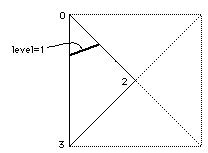### 19.5.4. Example

As a simple example consider one triangle with vertices labelled m1,m2 and m3 with heights 0, 2 and 3 respectively

Figure 19.4. Line permutations within contouring algorithm.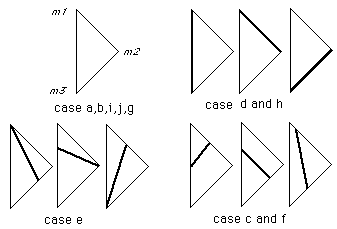To calculate where a contour line at a height of 1 should be drawn, it can be seen that this is case f described earlier. Level 1 intersects line segment m1-m2 half the way along and it intersects line segment m1-m3 one third of the way along. A line segment is drawn between these two points. Each rectangular mesh cell is treated this way.

### 19.5.5. Subroutine

An attempt is made at optimization by checking first to see if there are any contour levels within the present rectangle and second that there are some contour levels within the present triangle. The indices i and j are used to step through each rectangle in turn, k refers to each contour level and m to the four triangles in each rectangle.

Figure 19.5. Some of the notation used for identifying the rectangles and triangles in the subroutine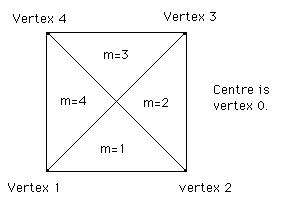Note that for large arrays the whole data array need not be stored in memory . Since the algorithm is a local one only requiring 4 points at a time, the data for each rectangle could be read from disk as required.

### 19.5.6. Example

Contour map and the following function

Figure 19.6. Function to be contoured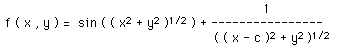Figure 19.7. Sample contour plot using CONREC algorithm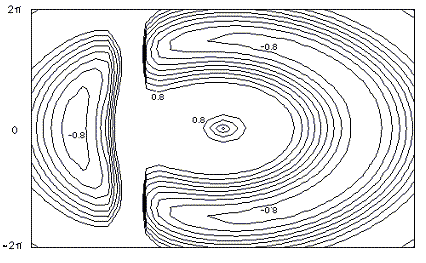Contouring algorithm

The sample contour plot itself

### 19.5.7. Note

On occasion users have reported gaps in their contour lines, this should of course never happen. There is however a pathological case that all local contouring algorithms suffer from (local meaning that they only use information in the immediate vicinity to determine the contour lines). The problem arises when all four vertices of a grid cell have the same value as the contour level under consideration. There are a number of strategies that can be employed to overcome this special event, the correct way is to consider a larger region in order to join up the contours on either side of the problem cell. CONREC doesn't do this and just leaves the cell without any contour lines thus resulting in a gap. This special case essentially never happens for real values data, it is most commonly associated with integer height datasets. The simplest solution is to offset the contour levels being drawn by a very small amount.